## Example Questions

### Example Question #9 : How To Find The Area Of A Trapezoid

A trapezoid has a base of length 4, another base of length s, and a height of length s. A square has sides of length s. What is the value of s such that the area of the trapezoid and the area of the square are equal?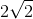Explanation:

In general, the formula for the area of a trapezoid is (1/2)(a + b)(h), where a and b are the lengths of the bases, and h is the length of the height. Thus, we can write the area for the trapezoid given in the problem as follows:

area of trapezoid = (1/2)(4 + s)(s)

Similarly, the area of a square with sides of length a is given by a2. Thus, the area of the square given in the problem is s2.

We now can set the area of the trapezoid equal to the area of the square and solve for s.

(1/2)(4 + s)(s) = s2

Multiply both sides by 2 to eliminate the 1/2.

(4 + s)(s) = 2s2

Distribute the s on the left.

4s + s2 = 2s2

Subtract s2 from both sides.

4s = s2

Because s must be a positive number, we can divide both sides by s.

4 = s

This means the value of s must be 4.

### Example Question #1 : Quadrilaterals

Find the area of a trapezoid given bases of length 1 and 2 and height of 2.Explanation:

To solve, simply use the formula for the area of a trapezoid. Thus,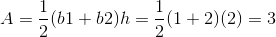### Example Question #2 : Quadrilaterals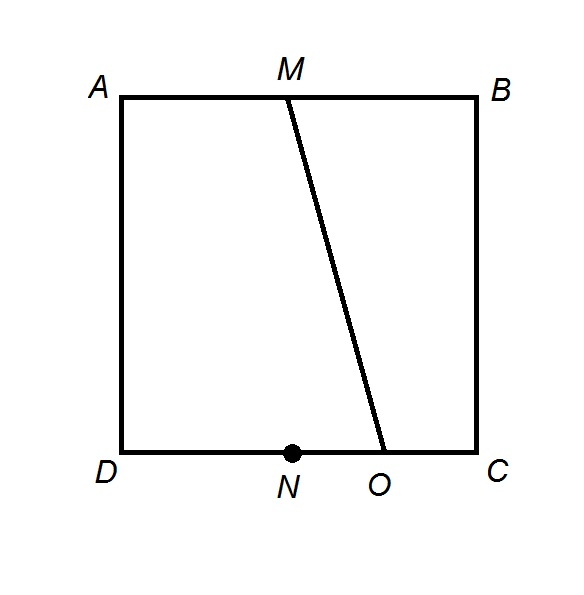The above figure shows Squareis the midpoint of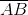is the midpoint of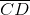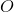is the midpoint of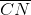. Construct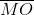If Squarehas area, what is the area of Quadrilateral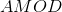?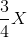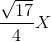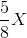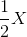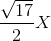Explanation:

Letbe the common sidelength of the square. The area of the square is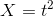.

Construct segment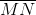. This divides the square into Rectangle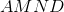and a right triangle. The dimensions of Rectangleare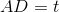and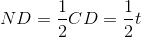.

The area of Rectangles the product of these dimensions: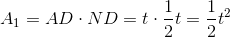The lengths of the legs of Right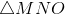are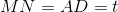and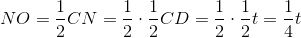The area of this right triangle is half the product of these lengths, or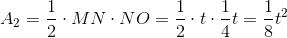This is seen below: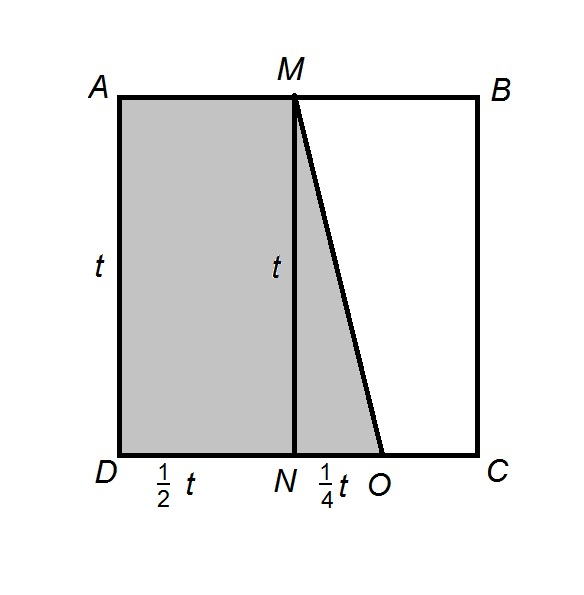The sum of these areas is the area of Quadrilateral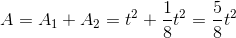.

Substitutingfor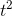, the area is.